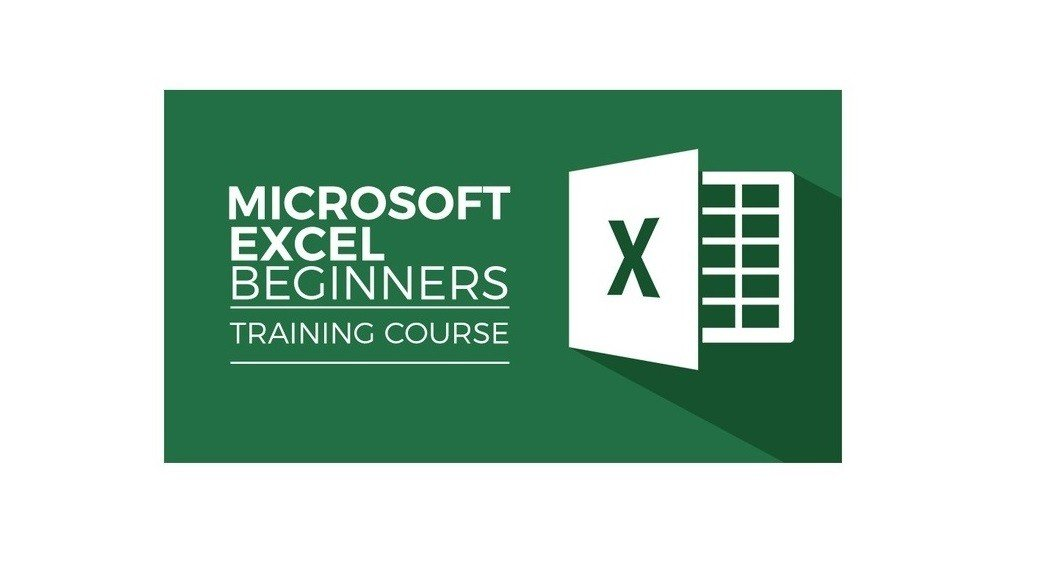Online Gurukul - हमारा उद्देश्य शिक्षित पूरा देश### Basics of Microsoft Excel Trending

Course Timeline:
Lesson 1 of Microsoft Excel is usually an introduction to the program and its basic features. Here are some of the things you might learn: Introduction to Excel: You will learn about what Excel is, its features, and why it is used. Excel Interface: You will be introduced to the Excel interface, including the ribbon, tabs, and groups, and how to navigate them. Worksheets and Workbooks: You will learn how to create and navigate worksheets and workbooks. You will also learn about the different parts of a worksheet, such as rows, columns, and cells. Entering Data: You will learn how to enter data into a cell, including text, numbers, and formulas. Editing Data: You will learn how to edit data in a cell, including changing the font, color, and size of text. Formatting: You will learn how to format cells, rows, and columns, including changing the font, color, and size of text, aligning text, and changing the background color of cells. Saving and Opening Workbooks: You will learn how to save your work in Excel, and how to open saved workbooks. Printing: You will learn how to print your Excel worksheets, including setting up the print area and adjusting the page orientation and margins. These are some of the basics you might cover in Lesson 1 of Microsoft Excel. By the end of this lesson, you should have a basic understanding of how to navigate and use Excel to enter and format data, and how to save and print your work.
Lesson 2 of Microsoft Excel usually focuses on using basic functions and formulas. Here are some of the things you might learn: Basic Functions: You will learn about basic functions in Excel such as SUM, AVERAGE, MIN, MAX, and COUNT. These functions are used to perform basic calculations on a range of cells. Formulas: You will learn how to create formulas in Excel. Formulas are used to perform more complex calculations and can include functions and mathematical operators. Cell References: You will learn about the different types of cell references in Excel, including relative, absolute, and mixed references. Cell references are used to refer to specific cells in a formula. AutoSum: You will learn how to use the AutoSum feature to quickly calculate the total of a range of cells. Conditional Formatting: You will learn how to use conditional formatting to highlight cells that meet specific criteria, such as cells that contain a certain value or are above or below a certain value. Sorting and Filtering: You will learn how to sort and filter data in Excel. Sorting allows you to arrange data in ascending or descending order, while filtering allows you to display only certain rows of data based on specific criteria. Charts: You will learn how to create charts in Excel. Charts are used to visualize data and make it easier to understand. These are some of the basics you might cover in Lesson 2 of Microsoft Excel. By the end of this lesson, you should have a basic understanding of how to use functions and formulas to perform calculations, how to format data using conditional formatting, and how to visualize data using charts.
Lesson 5 of Microsoft Excel usually focuses on working with large amounts of data and analyzing it using tools such as pivot tables and advanced filters. Here are some of the things you might learn: Advanced Filters: You will learn how to use advanced filters to filter data based on multiple criteria, as well as how to use wildcard characters to search for specific data. Data Analysis: You will learn how to use data analysis tools such as histograms, regression analysis, and data analysis add-ins to gain insights from large data sets. Pivot Table Calculations: You will learn how to perform advanced calculations within pivot tables, such as calculating running totals and percentages of totals. Power Query: You will learn how to use Power Query, a data connection and transformation tool in Excel, to combine, clean, and shape data from multiple sources. Conditional Logic: You will learn how to use conditional logic functions such as IF, AND, and OR to manipulate data and perform calculations. VBA Programming: You will learn the basics of VBA programming in Excel, which can be used to create custom macros and automate tasks. These are some of the advanced features you might cover in Lesson 5 of Microsoft Excel. By the end of this lesson, you should have a better understanding of how to work with large amounts of data, analyze it using various tools, and automate repetitive tasks using VBA programming.

## Course Reviews:

Average Rating 5
2 Ratings
Details
5 Stars 2
4 Stars 0
3 Stars 0
2 Stars 0
1 Stars 0
•By: Guru Abhishek Dwivedi
2 years ago

Very useful and very well presented

•By:
2 years ago

Use full

Trending

Trending